•••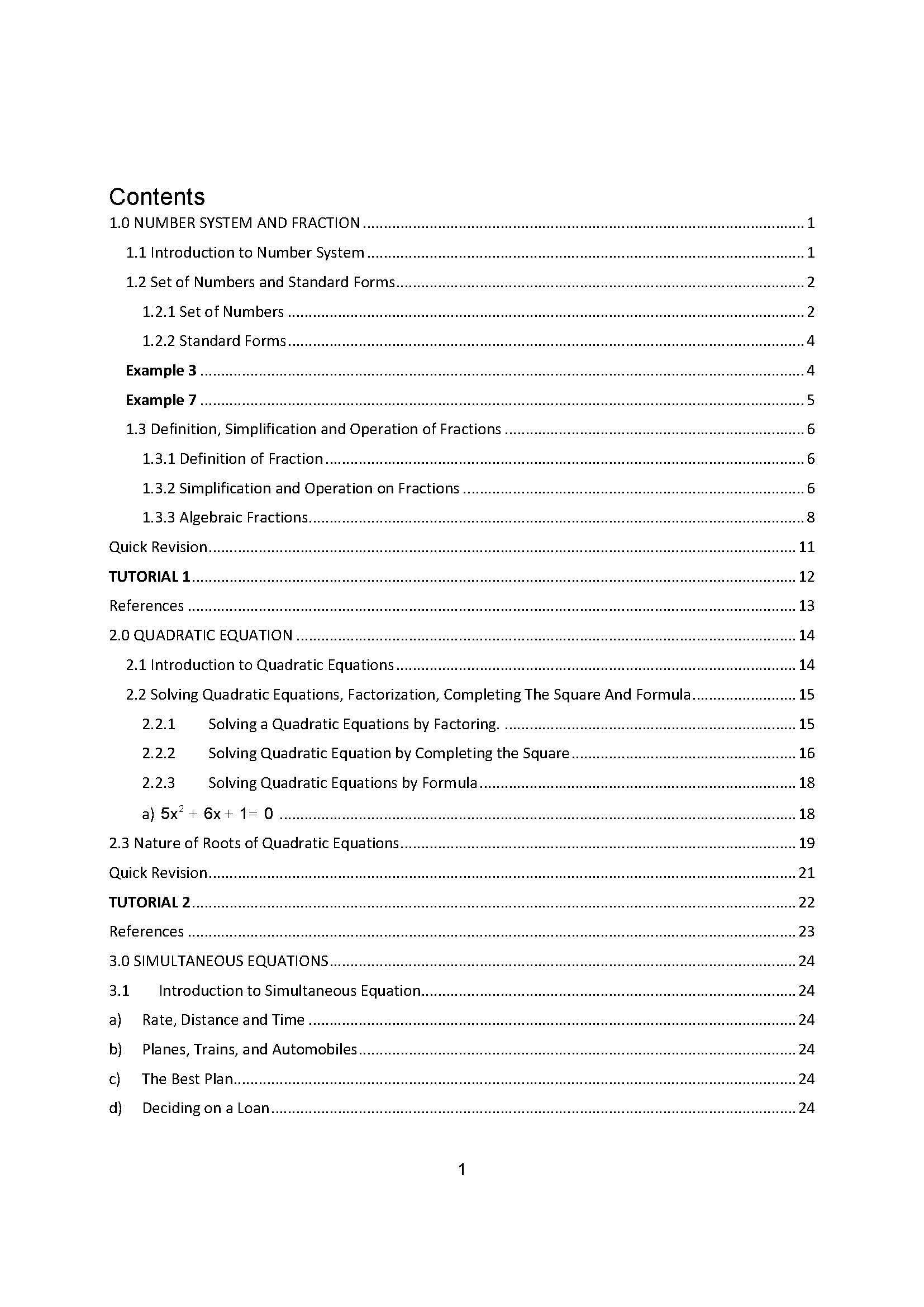•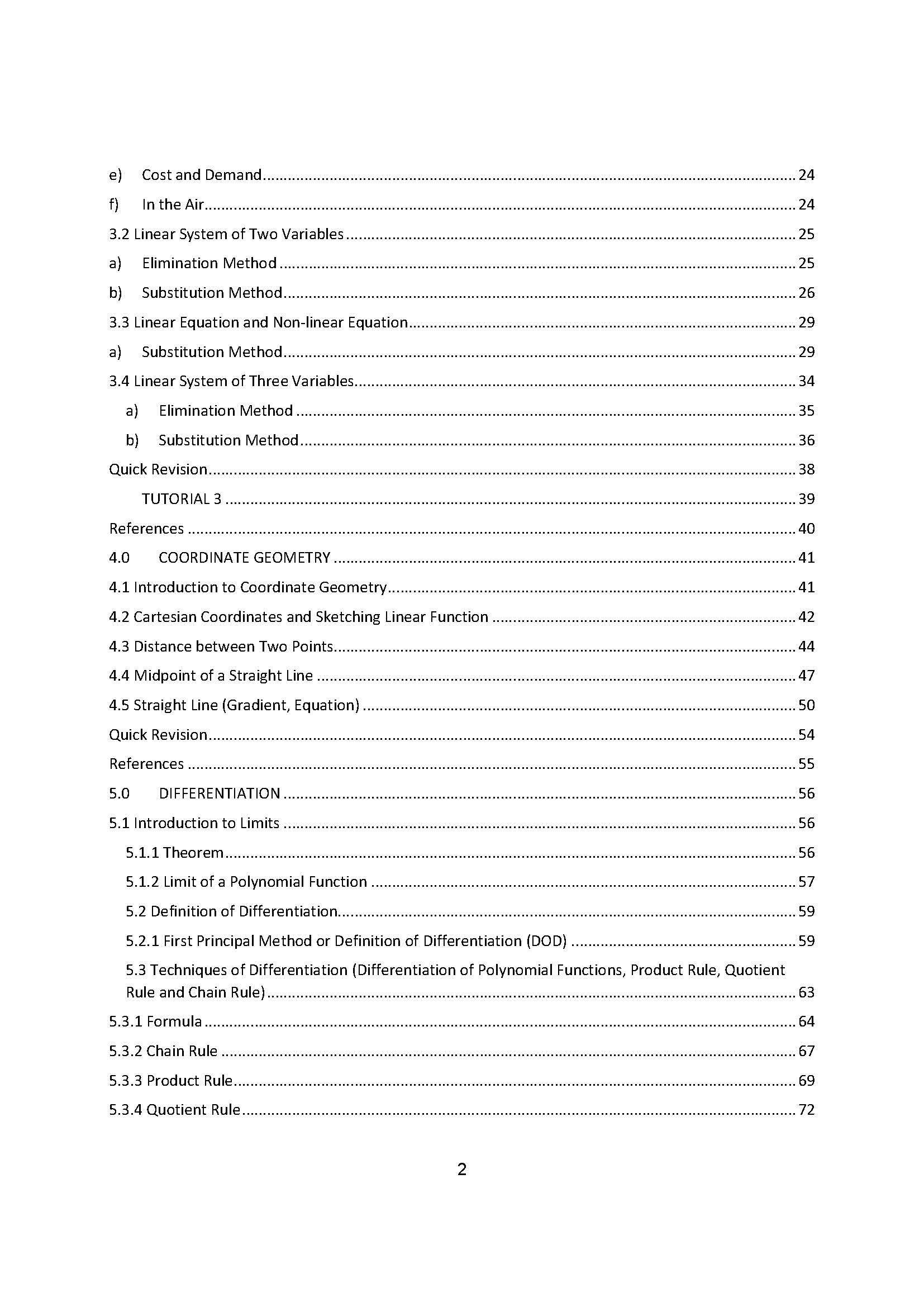•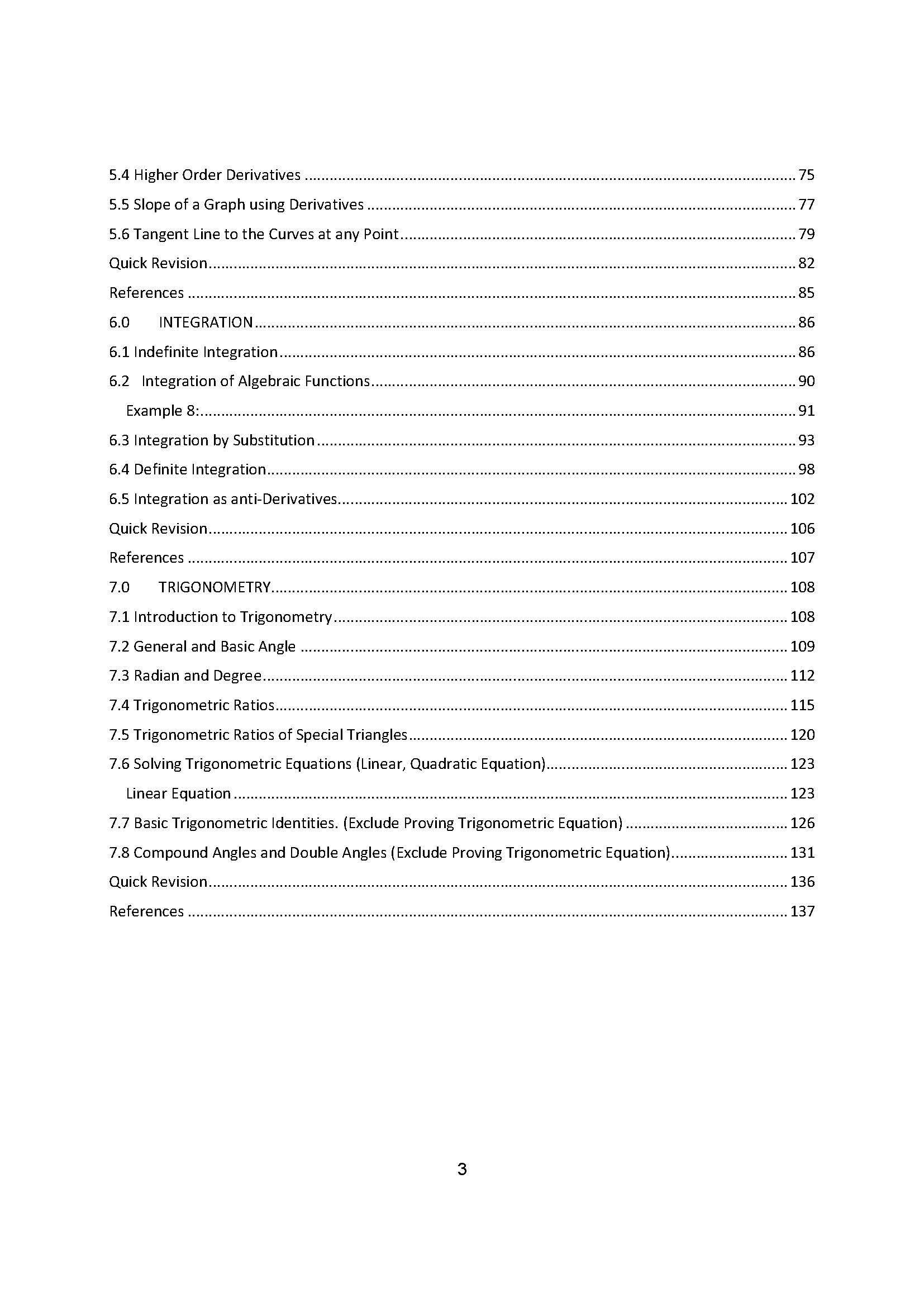•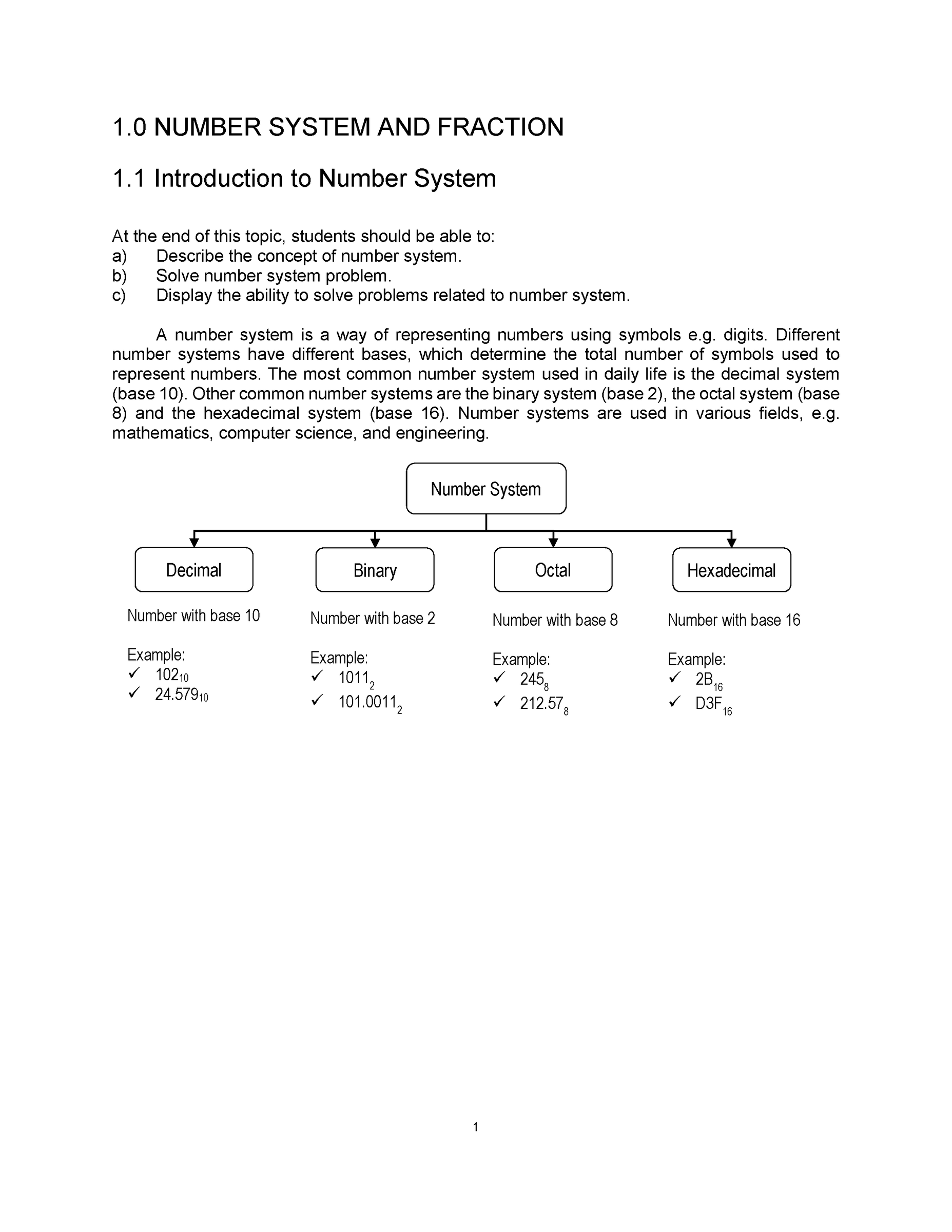•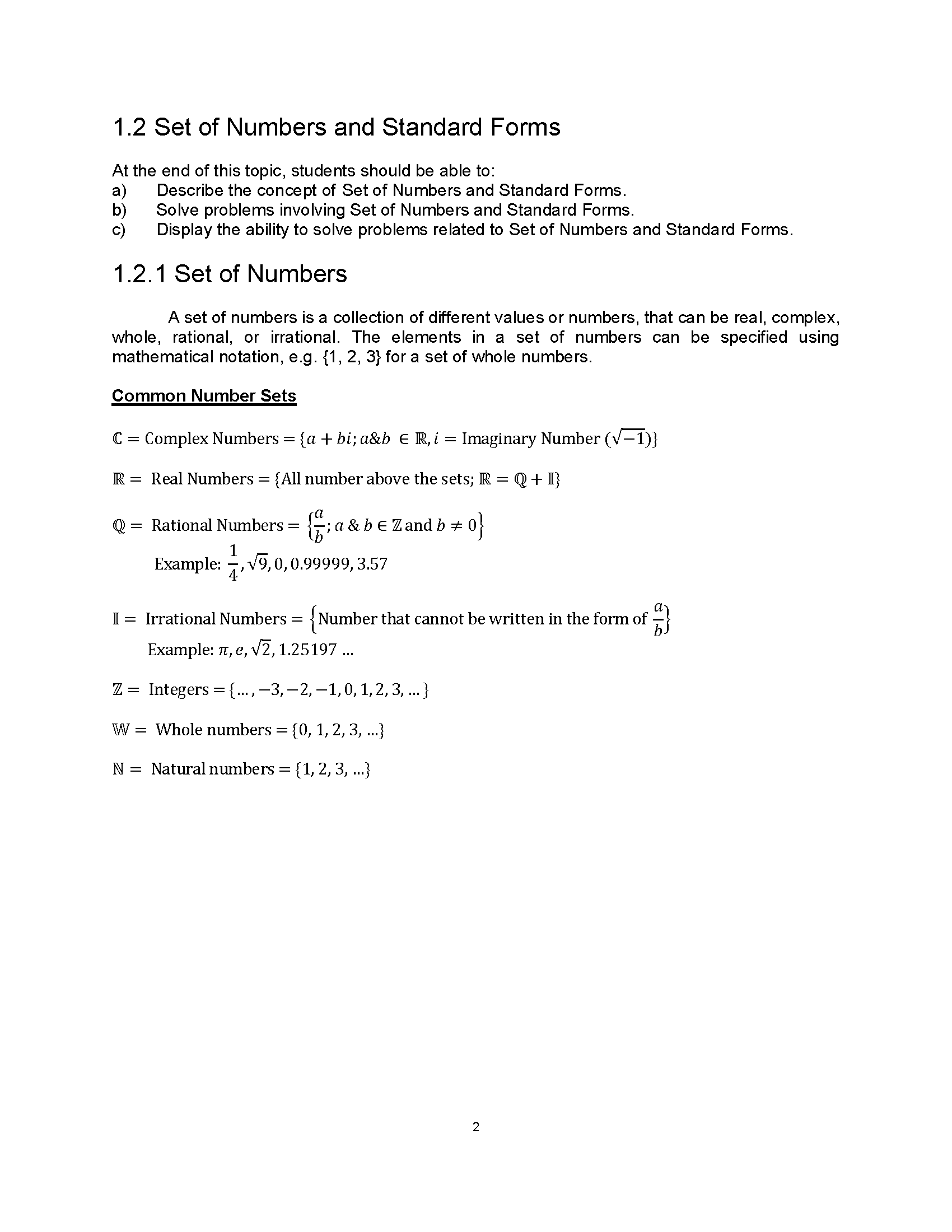•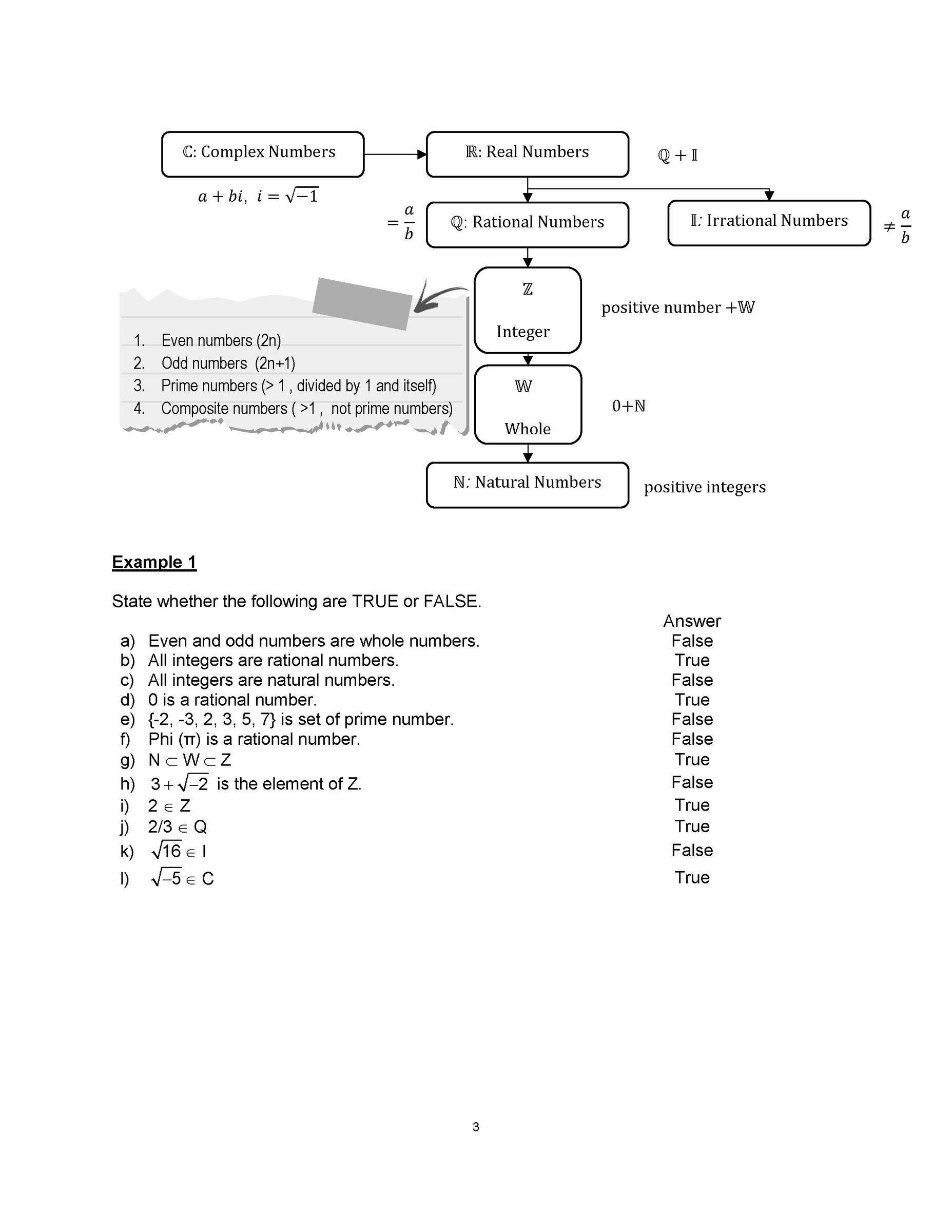•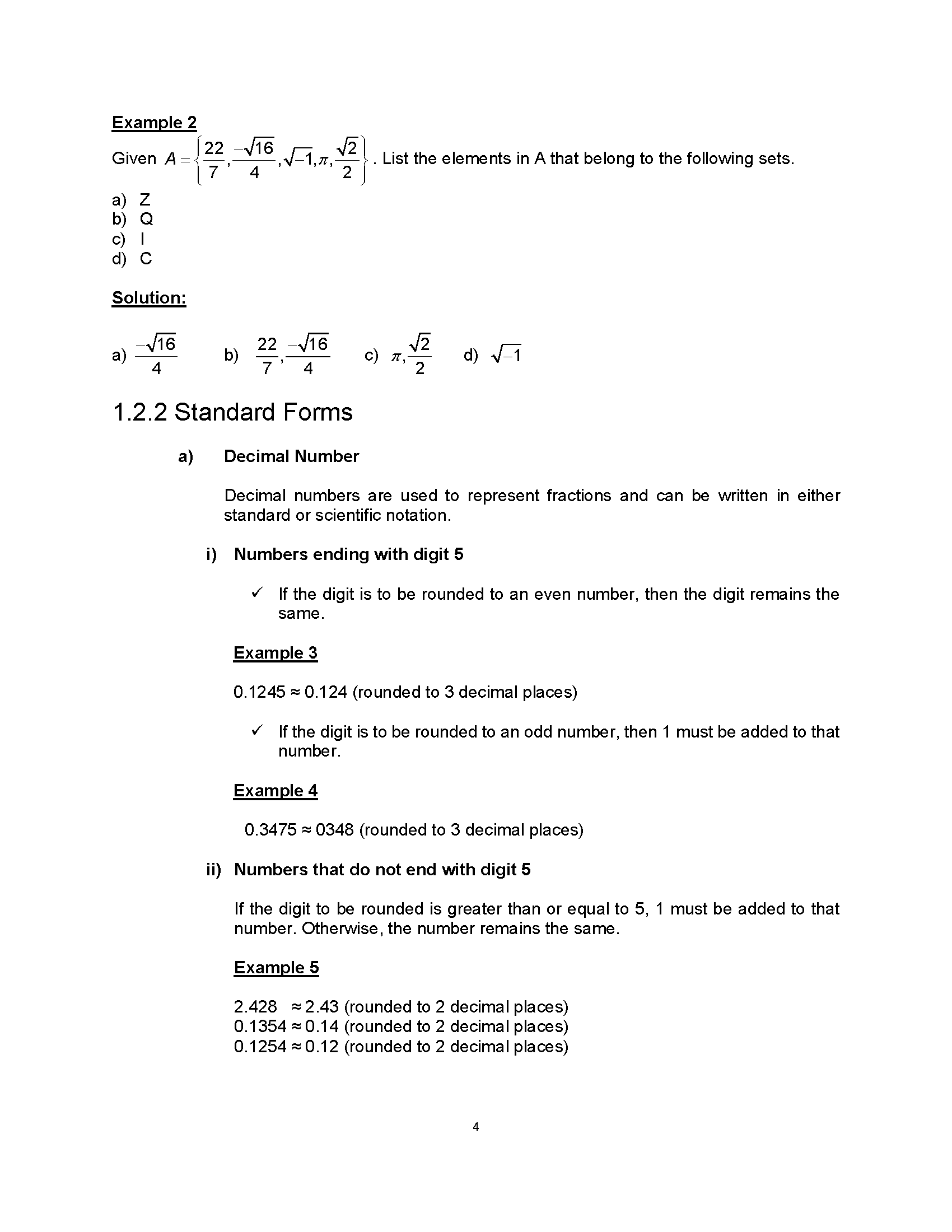# Basic Mathematics for Pre-Diploma Students

Regular price
RM 35.00
Sale price
RM 35.00
Regular price
RM 0.00

### Descriptions

This is suitable for self study.

Basic Mathematics for Pre-Diploma Students is specifically created to meet the demands of
pre-science students preparing for diploma and degree courses in higher education institutions. This seven-chapter book is designed for simple comprehension and appreciation of the topic.

Basic mathematics is where all mathematical concepts originated, forming the basis for many other academic fields, including physics, engineering, economics, and computer science. In this context, "basic" refers to everyday mathematical concepts and operations such as addition, subtraction, multiplication, and division.

Calculus, geometry, algebra, and trigonometry are a few examples of topics covered in fundamental mathematics. Together with the fundamental operations of addition, subtraction, multiplication, and division, arithmetic also deals with fractions, decimals, and percentages. Algebra focuses on the manipulation of variables and the solution of equations, whereas geometry deals with the features of shapes and their relationships. Whereas trigonometry concentrates on triangles and their properties, calculus emphasises the study of rates of change and integration.

The study of fundamental mathematics fosters the development of analytical abilities, logical reasoning, and problem-solving aptitudes—all of which are essential in many parts of life. It is essential to use mathematics in practical settings to understand ever-more-complex mathematical concepts.